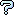All about flooble | fun stuff | Get a free chatterbox | Free JavaScript | Avatarsperplexus dot infoBasic Factoring (Posted on 2007-03-21)When math students are taught factoring, usually the first thing they learn are special factoring identities such as:

1: a˛-b˛= (a-b)(a+b)
2: ał-bł = (a-b)(a˛+ab+b˛)
3: ał+bł = (a+b)(a˛-ab+b˛)

Find a factorization of the expression x4+x2+1 using only those identities.

 Submitted by Brian Smith Rating: 3.0000 (1 votes) Solution: (Hide) Start with: x^6-1 = x^6-1 Apply (1) to the left side and (2) to the right side: (x^3-1)(x^3+1) = (x^2-1)(x^4+x^2+1) Next, apply (2) and (3) to the respective factors on the left side and apply (1) to (x^2-1) on the right side (x-1)(x^2+x+1)(x+1)(x^2-x+1) = (x-1)(x+1)(x^4+x^2+1) Since (x-1)(x+1) appears on each side, the factorization of x^4+x^2+1 is (x^2+x+1)(x^2-x+1) Check the comments for more ways to solve the problem, some of which include other equally basic factoring identites and methods.Comments: ( You must be logged in to post comments.)
 Subject Author DateAnother means of solution Mike C 2007-03-22 14:32:29solution xdog 2007-03-22 09:53:51An Attempt To The Solution K Sengupta 2007-03-22 00:42:24How's this? (Probable spoiler) Jer 2007-03-21 11:07:37Please log in:
 Login: Password: Remember me: Sign up! | Forgot password

 Search: Search body:
Forums (0)
Newest Problems
Random Problem
FAQ | About This Site
Site Statistics
New Comments (2)
Unsolved Problems
Top Rated Problems
This month's top
Most Commented On

Chatterbox:
Copyright © 2002 - 2019 by Animus Pactum Consulting. All rights reserved. Privacy Information